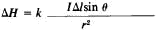Biot-Savart Law

(redirected from Biot-Savart's Law)

Biot-Savart law

A law of physics which states that the magnetic flux density (magnetic induction) near a long, straight conductor is directly proportional to the current in the conductor and inversely proportional to the distance from the conductor. The field near a straight conductor can be found by application of Ampère's law. The magnetic flux density near a long, straight conductor is at every point perpendicular to the plane determined by the point and the line of the conductor. Therefore, the lines of induction are circles with their centers at the conductor. Furthermore, each line of induction is a closed line. This observation concerning flux about a straight conductor may be generalized to include lines of induction due to a conductor of any shape by the statement that every line of induction forms a closed path.

McGraw-Hill Concise Encyclopedia of Physics. © 2002 by The McGraw-Hill Companies, Inc.
The following article is from The Great Soviet Encyclopedia (1979). It might be outdated or ideologically biased.

Biot-Savart Law

a law that determines the strength of the magnetic field created by an electric current. The Biot-Savart law was discovered by the French scientists J. B. Biot and F. Savart in 1820 and given a general formulation by P. Laplace. According to this law, a small segment of a conductor Δl along which a current of strength / is flowing creates—at a given point M in space, located at a distance r from the segment Δl (Δl << r)—a magnetic field of strengthHere θ is the angle between the direction of the current in segment / and the radius vector r, drawn from the segment to the observation point M, and k is the coefficient of proportionality, which depends on the choice of the system of units. In the centimeter-gram-second (cgs) system (Gauss), k=1/c, where c = 3 x 1010 cm/sec (the velocity of light in a vacuum); in the International System of Units (SI), k = l/4π.

The magnetic field strength ΔH is perpendicular to the plane P, which contains Δl and r, and its direction is determined by the auger rule: if the shaft of the auger (with a right-handed rifling) is turned from Δl toward r, then the auger advances in the direction of ΔH.

The total magnetic field strength ΔH created by a conductor with a current at point M is equal to the vector sum of the quantities ΔH, which are determined by all the elements Δ/ of the conductor. In particular, the strength H of a magnetic field at a distance d from a long (much greater than d), straight conducting wire along which a current of strength / is flowing is H = k·2I/d; in the center of a circle with radius R along which a current of strength / is flowing, H = k. 2πI/R; and on its axis, at a point remote from the plane of the circle at a distance d ≪ R, H = k·2π R2I/d3. On the axis of a solenoid of n turns, H = k·4πn/.

The Biot-Savart law can also be considered as a law that determines the magnetic induction ΔB ; in the cgs system, this requires that the expression for ΔH be multiplied by the magnetic permeability of the medium μ, whereas in the SI system, in addition, it must be multiplied by the magnetic permeability of a vacuum, μo = 4π10-7 henry/m.

G. IA. MIAKISHEV

Biot-Savart law

[¦byō sə¦vär ′lȯ]
(electromagnetism)
A law that gives the intensity of the magnetic field due to a wire carrying a constant electric current.
McGraw-Hill Dictionary of Scientific & Technical Terms, 6E, Copyright © 2003 by The McGraw-Hill Companies, Inc.
Mentioned in ?
References in periodicals archive ?
As was discovered well before the advent of relativity, the overall effect of currents on a moving charge can also be described completely by introducing the magnetic field B in the lab frame F and equating the Lorentz force to dp/dt The magnetic field B is calculated with Biot-Savart's Law. The main purpose of the derivation, which results in Eq.
An approximated analytical expression for each [[??].sub.i] is obtained using the Biot-Savart's law and simplifying the path of the ith current, [I.sub.i], considering that it follows a close trajectory formed by line segments, as given by (6).
The spatial distribution of the magnetostatic field is estimated superposing the magnetic induction numerically computed from the analytical expression of the magnetic field generated by each coil, obtained using the Biot-Savart's law and the current filament method.
For single or double circuit lines, two dimensional numerical analysis is sufficiently accurate in the majority of practical cases and the magnetic induction is computed by using Biot-Savart's law and the superposition principle .
In the particular case of plane symmetry assumption, the polarization plane is orthogonal to line conductors so that Biot-Savart's law can be used to compute the magnetic field.
Note that the same expressions are derived in  and  by using a multipole expansion of Biot-Savart's law.

Site: Follow: Share:
Open / Close